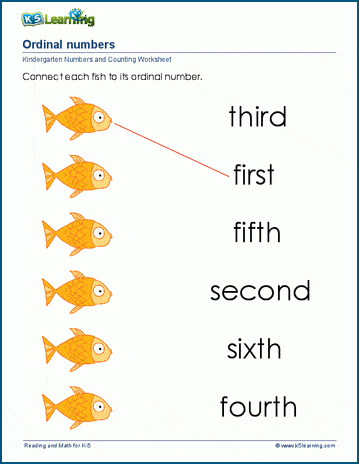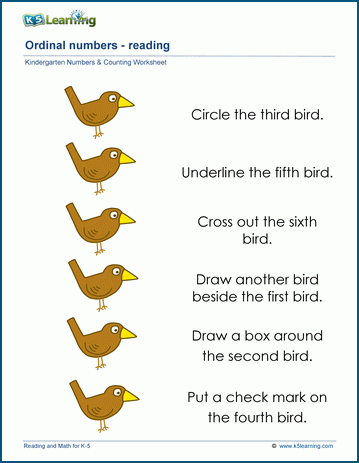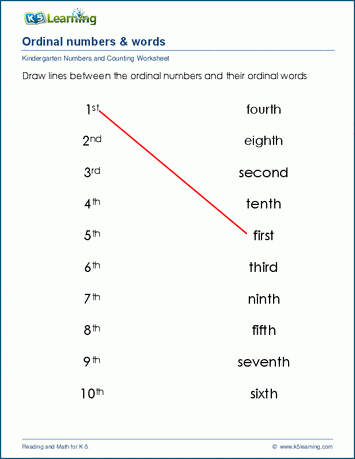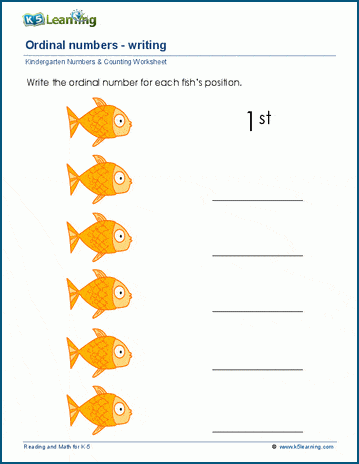# Ordinal Numbers Worksheets for Kindergarten

In our kindergarten numbers and counting section, we have a series of worksheets to help students practice the numbers and the words we use to define ordinal positions.

## Ordinal positions worksheets

Students practice matching the order of pictures with ordinal words (first, second, etc.) and ordinal numbers (1st, 2nd, etc.) in these worksheets.## Match ordinal numbers and ordinal words

Students match ordinal numbers between 1 to 10 with their corresponding ordinal words in these worksheets.## Writing ordinal numbers practice

These worksheets have students work on writing ordinal numbers and ordinal words.Become a Member

This content is available to members only.